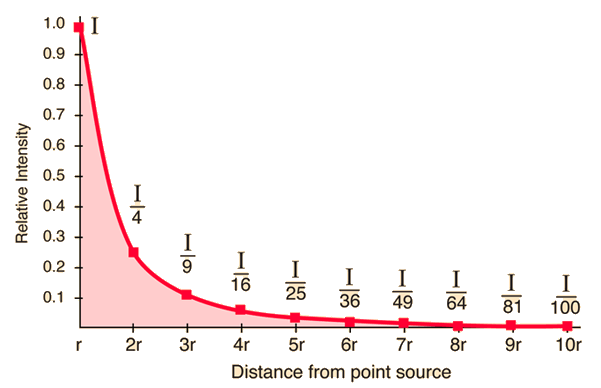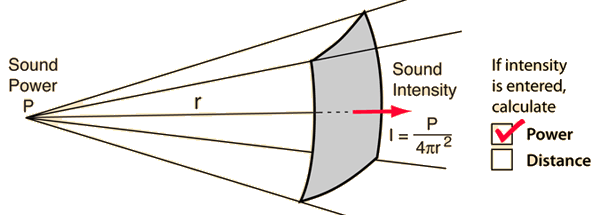# Inverse Square Law, Sound

The sound intensity from a point source of sound will obey the inverse square law if there are no reflections or reverberation. A plot of this intensity drop shows that it drops off rapidly.Calculation Intensity ratios Measurement example
 Other examples of inverse square law behavior: Gravity Electric Radiation Light
Index

Sound level measurement

Auditorium acoustics

 HyperPhysics***** Sound R Nave
Go Back

# Inverse Square Law Plot

A plot of the drop of sound intensity according to the inverse square law emphasizes the rapid loss associated with the inverse square law. In an auditorium, such a rapid loss is unacceptable. It is mitigated by the reverberation in a good auditorium. This plot shows the points connected by straight lines but the actual drop is a smooth curve between the points.Index

Sound level measurement

Auditorium acoustics

 HyperPhysics***** Sound R Nave
Go Back

# Sound Intensity from a Point Source

Sound from a point source obeys the inverse square law. It's intensity in decibels can be calculated by comparing the intensity to the threshold of hearing.

At a distance r = m = ft

from a point source of acoustic power P = watts

the sound intensity is x10^ watts/m2 = dB.Decibel definition Decibel calculation
 Calculating dB for distance ratios
 Estimating sound levels in decibels
Index

Sound level measurement

Auditorium acoustics

 HyperPhysics***** Sound R Nave
Go Back